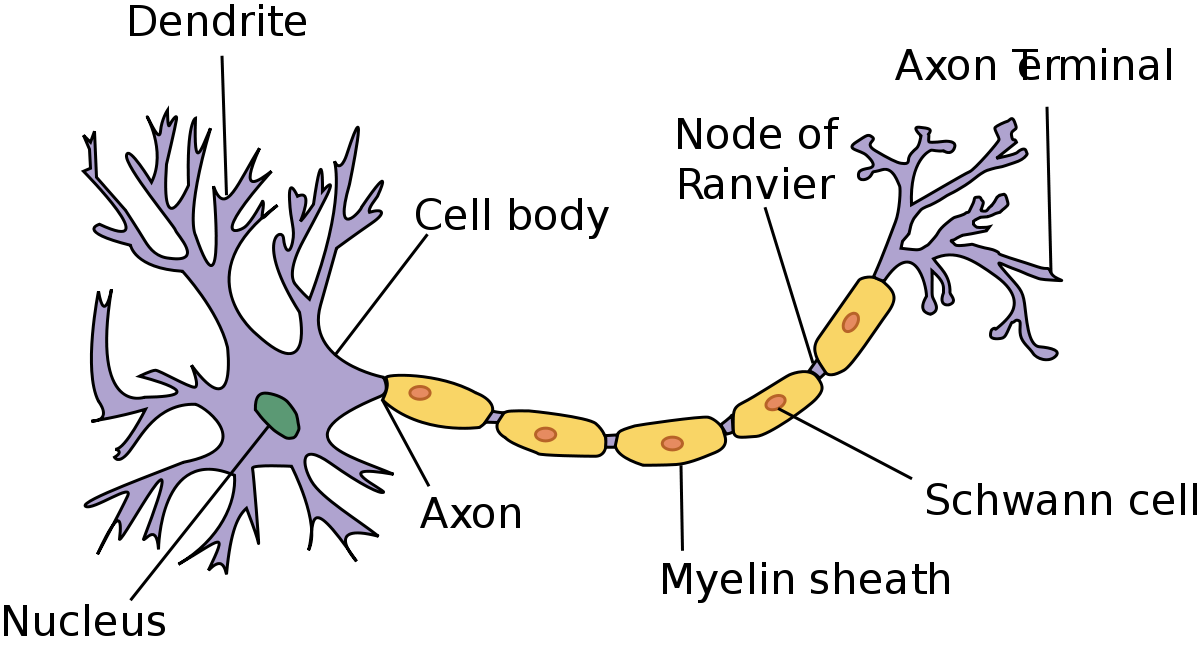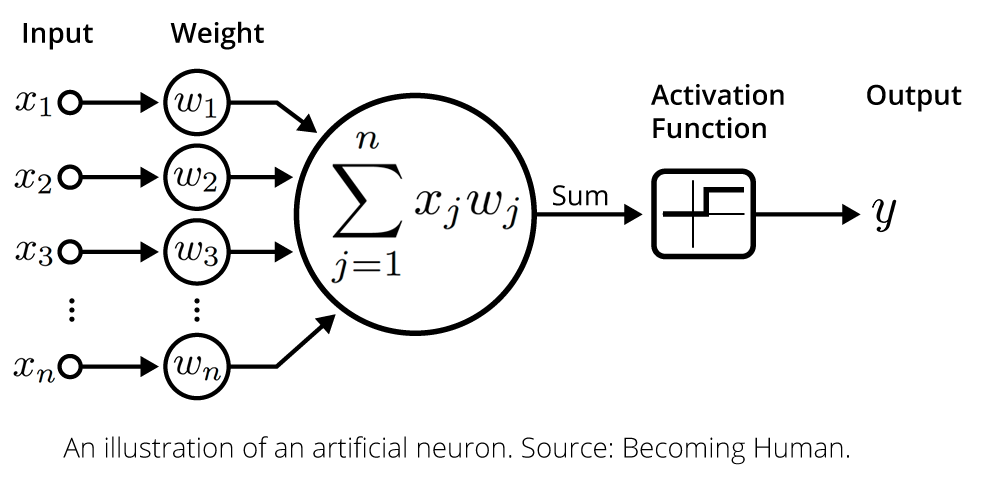# Understanding Neurons in Deep Learning

Hey - Nick here! This page is a free excerpt from my \$199 course Python for Finance, which is 50% off for the next 50 students.

Neurons are a critical component of any deep learning model.

In fact, one could argue that you can't fully understand deep learning with having a deep knowledge of how neurons work.

This article will introduce you to the concept of neurons in deep learning. We'll talk about the origin of deep learning neurons, how they were inspired by the biology of the human brain, and why neurons are so important in deep learning models today.

## What is a Neuron in Biology?

Neurons in deep learning were inspired by neurons in the human brain. Here is a diagram of the anatomy of a brain neuron:As you can see, neurons have quite an interesting structure. Groups of neurons work together inside the human brain to perform the functionality that we require in our day-to-day lives.

The question that Geoffrey Hinton asked during his seminal research in neural networks was whether we could build computer algorithms that behave similarly to neurons in the brain. The hope was that by mimicking the brain's structure, we might capture some of its capability.

To do this, researchers studied the way that neurons behaved in the brain. One important observation was that a neuron by itself is useless. Instead, you require networks of neurons to generate any meaningful functionality.

This is because neurons function by receiving and sending signals. More specifically, the neuron's `dendrites` receive signals and pass along those signals through the `axon`.

The `dendrites` of one neuron are connected to the `axon` of another neuron. These connections are called `synapses` - which is a concept that has been generalized to the field of deep learning.

## What is a Neuron in Deep Learning?

Neurons in deep learning models are nodes through which data and computations flow.

Neurons work like this:

• They receive one or more input signals. These input signals can come from either the raw data set or from neurons positioned at a previous layer of the neural net.
• They perform some calculations.
• They send some output signals to neurons deeper in the neural net through a `synapse`.

Here is a diagram of the functionality of a neuron in a deep learning neural net:Let's walk through this diagram step-by-step.

As you can see, neurons in a deep learning model are capable of having synapses that connect to more than one neuron in the preceding layer. Each synapse has an associated `weight`, which impacts the preceding neuron's importance in the overall neural network.

Weights are a very important topic in the field of deep learning because adjusting a model's weights is the primary way through which deep learning models are trained. You'll see this in practice later on when we build our first neural networks from scratch.

Once a neuron receives its inputs from the neurons in the preceding layer of the model, it adds up each signal multiplied by its corresponding weight and passes them on to an activation function, like this:The activation function calculates the output value for the neuron. This output value is then passed on to the next layer of the neural network through another synapse.

This serves as a broad overview of deep learning neurons. Do not worry if it was a lot to take in - we'll learn much more about neurons in deep learning throughout this course. For now, it's sufficient for you to have a high-level understanding of how they are structured in a deep learning model.

## Final Thoughts

In this tutorial, you had your first introduction to neurons in deep learning.

Here is a brief summary of what you learned:

• A quick overview of how neurons work in the human brain
• How neurons work in a deep learning model
• The different layers of neurons in a deep learning model
• The functionality of deep learning neurons
• How weights are applied to input signals within a neuron
• That activation functions are applied to the weighted sum of input signals to calculate a neuron's output value